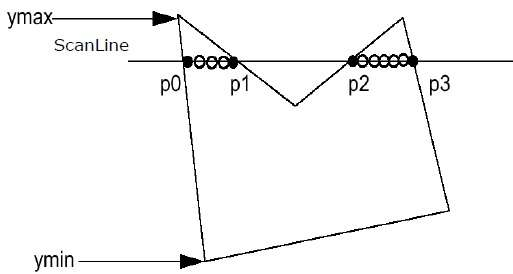World's most popular travel blog for travel bloggers.

# Explain the following with suitable examples:

, ,

(i) Windowing Transformations
(ii) Scan Line Polygon Fill Algorithm
(iii) Z-Buffer Algorithm
(iv) Sweep representations
(v) Simulating Accelerations in Computer Animations

## Windowing Transformations

Windowing is the process of transforming co-ordinates from one space to another. It is used when scaling and transforming the view of a program. For example: when you zoom into an image, the original image data is transformed to fill the current screen. This is done through an area referred to as the “window”.Diagram: showing how a set of co-ordinates can be transformed to the screen through a view window.
In the diagram above you can see windowing in action. The window acts as a go-between, rendering objects from infinite space into a finite area called the “screen”. Moving or scaling the window dimensions keeps the original co-ordinates of the image intact, but affects co-ordinates of the image being rendered.

## Scan Line Algorithm

This algorithm works by intersecting scanline with polygon edges and fills the polygon between pairs of intersections. The following steps depict how this algorithm works.
Step 1 − Find out the Ymin and Ymax from the given polygon.Step 2 − ScanLine intersects with each edge of the polygon from Ymin to Ymax. Name each intersection point of the polygon. As per the figure shown above, they are named as p0, p1, p2, p3.
Step 3 − Sort the intersection point in the increasing order of X coordinate i.e. (p0, p1), (p1, p2), and (p2, p3).
Step 4 − Fill all those pair of coordinates that are inside polygons and ignore the alternate pairs.

## Z-Buffering

An algorithm used in 3-D graphics to determine which objects, or parts of objects, are visible and which are hidden behind other objects. With Z-buffering, the graphics processor stores the Z-axis value of each pixel in a special area of memory called the Z-buffer .

Different objects can have the same x- and y-coordinate values, but with different z-coordinate values. The object with the lowest z-coordinate value is in front of the other objects, and therefore that's the one that's displayed.

An alternate algorithm for hiding objects behind other objects is called Z-sorting. The Z-sorting algorithm simply displays all objects serially, starting with those objects furthest back (with the largest Z-axis values). The Z-sorting algorithm does not require a Z-buffer, but it is slow and does not render intersecting objects correctly.